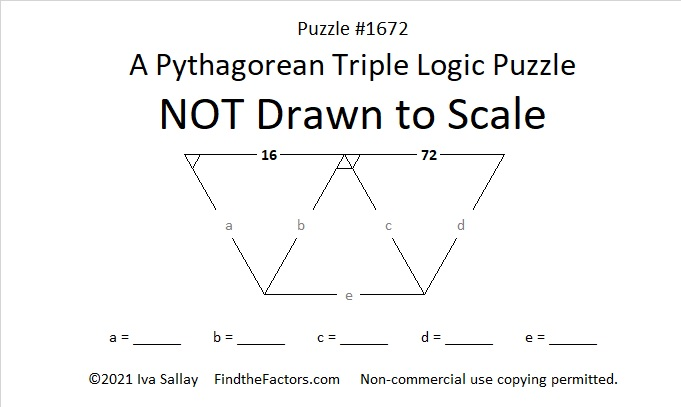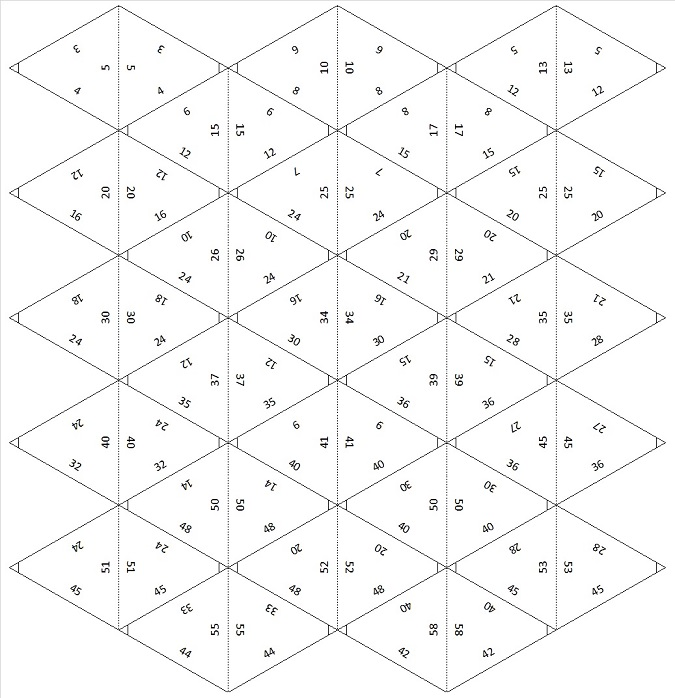# 1672 A Pythagorean Triple Puzzle with Only Two Clues

Contents

### Today’s Puzzle:

If you would like to try a Pythagorean Triple Puzzle that isn’t too difficult, I’ve got you covered with today’s puzzle:

PUZZLE DIRECTIONS: This puzzle is NOT drawn to scale. Although all of the angles may look like 60-degree angles, none of them are. The marked angles are 90 degrees. Lines that look parallel are NOT parallel. Although side lengths look equal, they are NOT equal. Most rules of geometry do not apply here. When drawn to scale, none of the triangles in this particular puzzle overlap, but that is not always the case for some of my other Pythagorean triple puzzles.

No geometry is needed to solve this puzzle. All that is needed is logic and the table of Pythagorean triples under the puzzle. The puzzle only uses triples in which each leg and each hypotenuse is less than 100 units long. The puzzle has only one solution.You can also print and cut out the triangle cards below to solve the puzzle.### Factors of 1672:

• Prime factorization: 1672 = 2 × 2 × 2 × 11 × 19, which can be written 1672 = 2³ × 11 × 19.
• 1672 has at least one exponent greater than 1 in its prime factorization so √1672 can be simplified. Taking the factor pair from the factor pair table below with the largest square number factor, we get √1672 = (√4)(√418) = 2√418.
• The exponents in the prime factorization are 3,1 and 1. Adding one to each exponent and multiplying we get (3 + 1)(1 + 1) (1 + 1) = 4 × 2 × 2 = 16. Therefore 1672 has exactly 16 factors.
• The factors of 1672 are outlined with their factor pair partners in the graphic below.### More About the Number 1672:

1672 is the difference of two squares in FOUR different ways:
419² – 417² = 1672,
211² – 207² = 1672,
49² – 27² = 1672, and
41² – 3² = 1672.
That last one means we are only 9 numbers away from the next perfect square, 1681.

From NumbersaPlenty, we learn that 1672 is a digitally powerful number:
1672 = 1¹ + 6⁴ + 7³ + 2⁵.

This site uses Akismet to reduce spam. Learn how your comment data is processed.